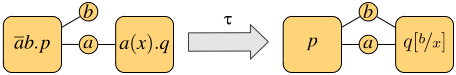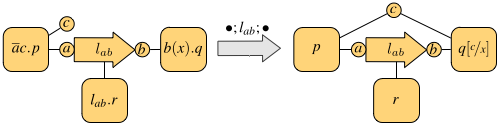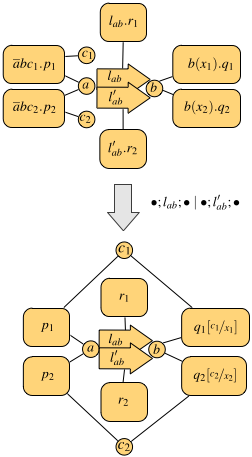ASCENS Project Blog
30Apr/13Off

## A network-aware extension of the pi-calculus

A key aspect of modern network architectures is the possibility of manipulating the network structure at run-time.  For instance, in Software Defined Networks  switches are instructed to install or remove forwarding rules at run-time by a controller machine; in IaaS Cloud Computing systems new nodes and links, equipped with a specific amount of storage, bandwidth, access rights..., can be allocated whenever needed.

Traditional process calculi, such as the pi-calculus, CCS and others, seem inadequate to describe these kinds of systems, because they abstract away from network details. In this post we give an overview of Network Conscious pi-calculus (NCPi)  [1,2], an extension of the pi-calculus with a natural notion of network. Following , we will first present a core version of NCPi, closer to the pi-calculus: we will recall the basic primitives of the pi-calculus and compare them with those of NCPi. Finally, we will present the "full-fledged" calculus.

## Base calculus

The pi-calculus models communication over channels. These are represented as pure names, that are entities characterized only by their identity. The novelty w.r.t. CCS is that communicated data are channels themselves, so processes can increase their communication capabilities during computation. The basic operations are output and input along channels: ab.p is a process that can send b along a and continue as p, and a(x).q can receive at a some datum that will replace x in the continuation q.

NCPi models communication over a network. There are two kinds of names: sites, atomic names of the form a representing network nodes, and links, structured names of the form lab, representing a link with name l from a to b. In addition to the same input and output primitives as the pi-calculus, NCPi also has a primitive to activate a linklab.p is a process that can provide a transportation service over lab to the environment and continue as p.

Both calculi have operators for executing two processes p and q in parallel, written p | q, and in a non-deterministic fashion, written p + q. The parallel execution may give rise to synchronizations, when the involved processes exchange messages. The synchronization mechanism is one major difference between the pi-calculus and NCPi. Both scenarios are exemplified in the following figures, where each process is depicted as a square with tentacles to its free names (only the relevant names are shown, which we assume to occur also in the subprocesses).###### Synchronization in the pi-calculus###### Synchronization in NCPi

The first figure shows the synchronization of the pi-calculus processes ab.p | a(x).q on the shared channel a: this gives rise to the observation tau and results in p and q sharing the channel b. In general, the pi-calculus only allows communication on shared channels, whereas NCPi is able to model remote communications. In fact, the second figure shows two processes that do not share output and input sites, but there is a process executing in parallel that provides a link between them, thus enabling the communication. The resulting observation is the link traversed by the datum. In general, a single communication may use a chain of links of arbitrary length.

NCP inherits the mechanism for creating and communicating new resources from the pi-calculus: fresh, unguessable sites and links are declared via the restriction operator (x)p, which binds x in p; communication of bound names enlarges their scope to the receiving process, which can use such names from then on. This is the mechanism that captures mobility.

## Concurrent calculus

The calculus presented so far has an interleaving semantics, meaning that the behavior of parallel processes is given by their interleaved execution. This implicitly assumes the existence of a central arbiter who decides in which order resources should be accessed. However, such assumption can be considered inadequate for distributed systems with partially asynchronous behavior. We propose a version of NCPi which is closer to "real-life" networks. Its main features are:

• the output operator is of the form abc.p, modeling the emission of a packet from a with destination b and payload c.
• the semantics is concurrent, in the sense that many transmissions can be observed at the same time.

Concurrency in the semantics allows the associated behavioral equivalence to distinguish a parallel process from its interleaving counterpart (technically speaking, this avoids the usual counterexample where ab.a'(x) + a'(x).ab cannot simulate ab | a'(x) whenever a is identified with a', because the two processes are not equivalent in the first place). Indeed, it can be shown that such equivalence is preserved by all NCPi operators.

An example of parallel execution is depicted in the following figure.The top process has two links between a and b (for instance, one could be wired and the other one wireless). This enables the two processes on the left to transmit their data, both from a and addressed to b, at the same time. In fact, the resulting observation is made of two communications, each using one of the links from a to b, and both input variables are instantiated with actual values in the continuation (the bottom process).

 U. Montanari and M. Sammartino. Network conscious -calculus: A concurrent semantics. Electr. Notes Theor. Comput. Sci., 286:291–306, 2012. Available at http://www.di.unipi.it/ sammarti/papers/mfps28.pdf.

 U. Montanari and M. Sammartino. A network-conscious pi-calculus and its coalgebraic semantics. Theor. Comput. Sci. To Appear.